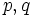# Centralizer product theorem for elementary abelian group

## Statement

Suppose$p,q$ are distinct primes. Suppose$P$ is an elementary abelian$p$-group, and$Q \le \operatorname{Aut}(P)$ is an abelian$q$-group that is not cyclic. Let$x_1, x_2, \dots, x_n$ be an enumeration of the non-identity elements of$Q$. Then, we have:$P = C_P(x_1)C_P(x_2) \dots C_P(x_n)$.

where$C_P(x_i)$ denotes the set of fixed points in$P$ of the automorphism$x_i$.

## Related facts

### Generalizations

• Centralizer product theorem: The same result, except that$P$ is now an arbitrary$p$-group, rather than an elementary abelian group.

## Facts used

1. Maschke's averaging lemma

## Proof

Given: Primes$p \ne q$. An elementary abelian$p$-group$P$, a non-cyclic abelian$q$-subgroup$Q$ of$\operatorname{Aut}(P)$.$x_1, x_2, \dots, x_n$ are the non-identity elements of$Q$.

To prove:$P = C_P(x_1)C_P(x_2) \dots C_P(x_n)$.

Proof:

1. By fact (1), viewing$P$ as a vector space over the field of$p$ elements and$Q$ as a group acting on this vector space, we can decompose$P$ as a direct sum of irreducible$Q$-modules, say$P_1, P_2, \dots, P_r$.
2. Let$Q_i$ be the pointwise stabilizer of$P_i$ in$Q$. Then,$Q/Q_i$ is cyclic:$Q/Q_i$ is an abelian group of automorphisms of the vector space$P_i$, and$P_i$ is irreducible. This forces$Q/Q_i$ to be cyclic.
3. Each$Q_i$ is a nontrivial subgroup of$Q$: This follows from the previous step and the fact that$Q$ isn't cyclic.
4. For each$i$, pick a non-identity element$x$ of$Q_i$. Then,$C_P(x)$ contains$P_i$: This follows from the definition of$Q_i$.
5.$P = \sum C_P(x_i)$: Each$P_i$ is contained in$C_P(x)$ for some non-identity element$x$. Thus,$P$ is the sum of all$C_P(x)$,$x$ ranging over the non-identity elements of$P$.

Reverting to multiplicative notation yields the result.